Geometry 2013-2014 SSC (Marathi Semi-English) 10th Standard [इयत्ता १० वी] Question Paper Solution

Geometry
Date: March 2014

 1 | Solve any five sub-questions:
 1.1

In the following figure RP: PK= 3:2, then find the value of A(ΔTRP):A(ΔTPK).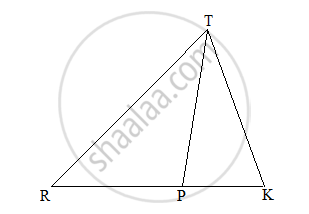Concept: Properties of Ratios of Areas of Two Triangles
Chapter: [0.01] Similarity
 1.2

If two circles with radii 8 cm and 3 cm, respectively, touch internally, then find the distance between their centers.

Concept: Touching Circles
Chapter: [0.03] Circle
 1.3

If the angle θ = -60° , find the value of sinθ .

Concept: Trigonometric Ratios of Complementary Angles
Chapter: [0.06] Trigonometry
 1.4

Find the slope of the line passing through the points A(2,3) and B(4,7).

Concept: Slope of a Line
Chapter: [0.04] Co-ordinate Geometry
 1.5

The radius of a circle is 7 cm. find the circumference of the circle.

Concept: Circumference of a Circle
Chapter: [0.07] Mensuration [0.07] Mensuration
 1.6

If the sides of a triangle are 6 cm, 8 cm and 10 cm, respectively, then determine whether the triangle is a right angle triangle or not.

Concept: Right-angled Triangles and Pythagoras Property
Chapter: [0.02] Pythagoras Theorem
 2 | Solve any four sub-questions:
 2.1

In the figure given below, Ray PT is bisector of ∠QPR. If PQ = 5.6 cm, QT = 4 cm and TR = 5 cm, find the value of x .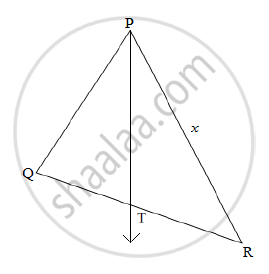Concept: Similarity of Triangles
Chapter: [0.01] Similarity
 2.2

In the following figure, Q is the center of the circle. PM and PN are tangents to the circle. If ∠MPN = 40° , find ∠MQN.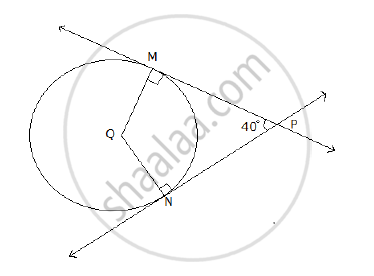Concept: Number of Tangents from a Point on a Circle
Chapter: [0.03] Circle [0.03] Circle
 2.3

Write the equation 2x - 3y - 4 = 0 in the slope intercept form. Hence, write the slope and y-intercept of the line.

Concept: Intercepts Made by a Line
Chapter: [0.04] Co-ordinate Geometry
 2.4

If cosθ=1/sqrt(2), where θ is an acute angle, then find the value of sinθ.

Concept: Trigonometric Ratios of Complementary Angles
Chapter: [0.06] Trigonometry
 2.5

If (4,-3) is a point on the line AB and slope of the line is (-2), write the equation of the line AB.

Concept: General Equation of a Line
Chapter: [0.04] Co-ordinate Geometry
 2.6

Draw a tangent at any point ‘P’ on the circle of radius 3.5 cm and centre O.

Concept: Construction of Tangent to the Circle from the Point on the Circle
Chapter: [0.05] Geometric Constructions
 3 | Solve any three sub-questions:
 3.1

In a triangle ABC, line l || Side BC and line l intersects side AB and AC in points P and Q, respectively. Prove that: "AP"/"BP"="AQ"/"QC"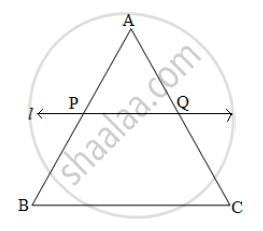Concept: Similarity of Triangles
Chapter: [0.01] Similarity
 3.2

In figure, ΔABC is an isosceles triangle with perimeter 44 cm. The base BC is of length 12 cm. Side AB and side AC are congruent. A circle touches the three sides as shown in the figure below. Find the length of the tangent segment from A to the circle.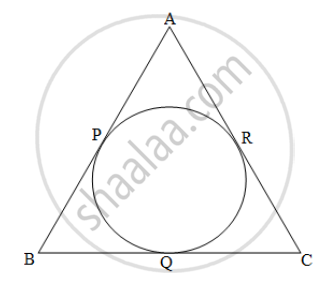Concept: Circumference of a Circle
Chapter: [0.07] Mensuration [0.07] Mensuration
 3.3

Draw tangents to the circle with center ‘C’ and radius 3.6 cm, from a point B at a distance of 7.2 cm from the center of the circle.

Concept: Construction of Tangents to a Circle
Chapter: [0.05] Geometric Constructions
 3.4

Prove that:

sec2θ + cosec2θ = sec2θ x cosec2θ

Concept: Trigonometric Identities
Chapter: [0.06] Trigonometry
 3.5

Write the equation of each of the following lines:

1. The x-axis and the y-axis.
2. The line passing through the origin and the point (-3, 5).
3. The line passing through the point (-3, 4) and parallel to X-axis.
Concept: General Equation of a Line
Chapter: [0.04] Co-ordinate Geometry
 4 | Solve any two sub-questions:
 4.1

From the top of a lighthouse, an observer looks at a ship and finds the angle of depression to be 60° . If the height of the lighthouse is 90 meters, then find how far is that ship from the lighthouse? (√3 = 1.73)

Concept: Heights and Distances
Chapter: [0.06] Trigonometry
 4.2

Prove that the “the opposite angles of the cyclic quadrilateral are supplementary”.

Concept: Cyclic Properties
Chapter: [0.03] Circle
 4.3

The sum of length, breadth and height of a cuboid is 38 cm and the length of its diagonal is 22 cm. Find the total surface area of the cuboid.

Concept: Surface Area of a Combination of Solids
Chapter: [0.07] Mensuration
 5 | Solve any two sub-questions:
 5.1

In triangle ABC, ∠C=90°. Let BC= a, CA= b, AB= c and let 'p' be the length of the perpendicular from 'C' on AB, prove that:

1. cp = ab

2. 1/p^2=1/a^2+1/b^2

Concept: Right-angled Triangles and Pythagoras Property
Chapter: [0.02] Pythagoras Theorem
 5.2

Construct the circumcircle and incircle of an equilateral triangle ABC with side 6 cm and centre O. Find the ratio of radii of circumcircle and incircle.

Concept: Division of a Line Segment
Chapter: [0.04] Co-ordinate Geometry [0.05] Geometric Constructions
 5.3

There are three stair-steps as shown in the figure below. Each stair step has width 25 cm, height 12 cm and length 50 cm. How many bricks have been used in it, if each brick is 12.5 cm x 6.25 cm x 4 cm?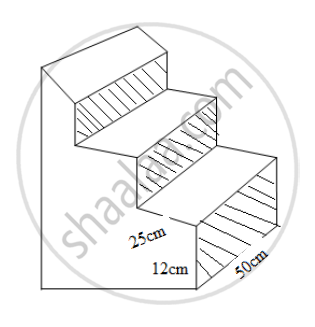Concept: Heights and Distances
Chapter: [0.06] Trigonometry

Request Question Paper

If you dont find a question paper, kindly write to us

View All Requests

Submit Question Paper

Help us maintain new question papers on Shaalaa.com, so we can continue to help students

only jpg, png and pdf files

Maharashtra State Board previous year question papers 10th Standard [इयत्ता १० वी] Geometry with solutions 2013 - 2014

Maharashtra State Board 10th Standard [इयत्ता १० वी] Geometry question paper solution is key to score more marks in final exams. Students who have used our past year paper solution have significantly improved in speed and boosted their confidence to solve any question in the examination. Our Maharashtra State Board 10th Standard [इयत्ता १० वी] Geometry question paper 2014 serve as a catalyst to prepare for your Geometry board examination.
Previous year Question paper for Maharashtra State Board 10th Standard [इयत्ता १० वी] Geometry-2014 is solved by experts. Solved question papers gives you the chance to check yourself after your mock test.
By referring the question paper Solutions for Geometry, you can scale your preparation level and work on your weak areas. It will also help the candidates in developing the time-management skills. Practice makes perfect, and there is no better way to practice than to attempt previous year question paper solutions of Maharashtra State Board 10th Standard [इयत्ता १० वी].

How Maharashtra State Board 10th Standard [इयत्ता १० वी] Question Paper solutions Help Students ?
• Question paper solutions for Geometry will helps students to prepare for exam.
• Question paper with answer will boost students confidence in exam time and also give you an idea About the important questions and topics to be prepared for the board exam.
• For finding solution of question papers no need to refer so multiple sources like textbook or guides.